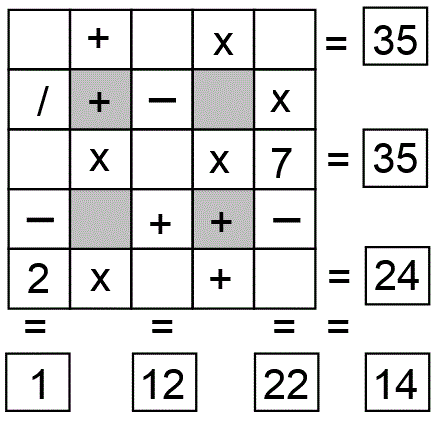Select Page

Each month, a new set of puzzles will be posted.  Come back next month for the solutions and a new set of puzzles, or subscribe to have them sent directly to you.

## EQUATE+2 Puzzle

Each row, column & diagonal is an equation and you use the numbers 1 to 9 to complete the equations. Each number can be used only once. Two numbers have been provided to get you started. Find the remaining seven numbers that satisfies all the resulting equations.
Note – multiplication (x) & division (/) are performed before addition (+) and subtraction (-).## EQUATE+0 Puzzle

Each row, column & diagonal is an equation and you use the numbers 1 to 9 to complete the equations. Each number can be used only once. No numbers have been provided to get you started. Find the remaining nine numbers that satisfies all the resulting equations.
Note – multiplication (x) & division (/) are performed before addition (+) and subtraction (-).## Feedback

There are more than one way of doing these puzzles and may well be more than one answer.  Please let me and others know what alternatives you find by commenting below.  We also welcome general comments on the subject and any feedback you'd like to give.

If you have a question that needs a response from me or you would like to contact me privately, please use the contact form.

## Get more puzzles!

If you've enjoyed doing the puzzles, consider ordering the books;

• Book One - 150+ of the best puzzles
• Book Two - 200+ with new originals and more of your favourites

Both in a handy pocket sized format.   Click here for full details.

## MATCHSTICK Challenge:

Here is a figure with 11 matchsticks. Move two matchsticks to make eleven squares (of any size). All matchsticks must be used.

Solution:## MIND-Xpander (Circles in a Rectangle Puzzle – Level 2):

The diagram below shows two touching circles with equal radius and completely inside a rectangle as shown below. The circles have the same radius, are not over-lapping and each touch two sides of the rectangle, as shown. What are the radii of the circles if the dimensions of the rectangle are 48cm by 54cm? Note: The diagram is not drawn to scale.

Solution:a² + b² = c²
(48 – 2R)² + (54 – 2R)² = (2R)²
(2304 – 192R + 4R²) + (2916 – 216R + 4R²) = 4R²
4R² + 4R² – 192R – 216R + 2304 + 2916 = 4R²
8R² – 4R² – 408R + 5220 = 0
4R² – 408R + 5220 = 0
R² – 102R + 1305 = 0 & then, (R -15) (R – 87) = 0
Only R – 15 = 0 qualify and therefore, R=15

Checking for R = 15 : (15)² – (102 * 15) + 1305 = 0
225 – 1530 + 1305 = 0
0 = 0

Answer: The maximum radius of two identical circles which can be “completely put inside a rectangle of dimensions of 48cm by 54cm” without overlapping is 15cm.